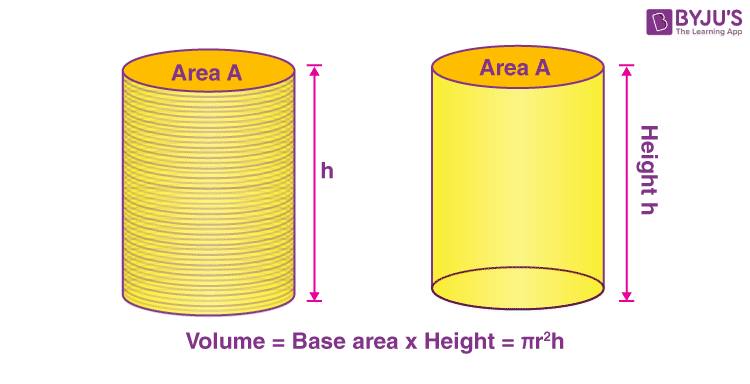# Volume Of Cube, Cuboid And Cylinder

Cube, cuboid and cylinder are three-dimensional shapes having circular or rectangular faces. A cube or a cuboid has six faces and eight vertices, on the other hand, a cylinder has two circular faces joined by a curved surface.

The area of all the faces of a cube is the same as they are all squares. Now, think of a scenario where we need to calculate the amount of sugar that can be accommodated in a cubical, cuboidal or a cylindrical box. In other words, we mean to calculate the capacity of the box.

The capacity of a cubical, cuboidal or a cylindrical box is basically equal to the volume of cube, cuboid or the cylinder used. The volume of a three-dimensional shape, in general, is equal to the amount of space occupied by that shape. Let us calculate the volume of some common three-dimensional shapes:

## Volume of cuboid

Consider a cuboid having Length, Width and Height to be l, b, h respectively. Then,

The Volume of a cuboid is given by the product of its dimensions.

Volume = l × b × h

## Volume of the cube

A Cuboid having all the dimension to be of equal length is known to be a cube. Consider the dimension of a cube to be “a” then the volume of a cube is given as-

Volume of a cube = a × a × a= a3

Try outCube Calculator

#### For More Information On Volumes of Cubes and Cuboid, Watch The Below Video:## Volume of a cylinder

A cylinder can be seen as a set of circular disks that are stacked on one another. In order to calculate the space occupied by a cylinder, we calculate the space occupied by each disk and then add them up. Thus, the volume of the cylinder can be given by the product of the area of base and height. There are many other properties of Cylinder.Consider a cylinder having a base radius of ‘r’ and height to be ‘h,’ thus the volume of a cylinder is given by-

Volume of a cylinder = (area of base) × h

= (π r2) h

= π r2 h

## Solved Problems

Question 1:Calculate the radius of the base of a cylindrical container of volume 220 m3. The height of the cylindrical container is 70 m. (Take pi = 22/7)

Solution: Volume of a cylinder = (area of base) × height of a cylinder

Area of base = (Volume of cylinder)/ (height of cylinder) = 220/70 m2

22/7 r2 = 220/70

r2 = 1

r = 1

Question 2: Calculate the amount of air that can be accumulated in a cube of side 3 m.

Solution: Amount of air that can be accumulated in a cube = capacity of the cube = volume of cube

Volume of cube = a3 = 3 × 3 × 3 = 27 m3

Thus, the amount of air accumulated in a cube of 3 m side = 27 m3

Stay tuned with BYJU’S to learn more about volume and areas, volume of combination of solids and many other topics.

Test your Knowledge on Volume Of Cube, Cuboid And Cylinder